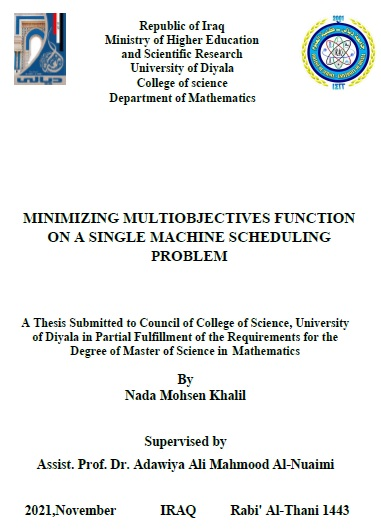## رسالة ماجستير / ندى محسن

اعلام المكتبة المركزية / المهندس مهند

Abstract

In this thesis, simultaneous multi criteria scheduling problem on

single machine is presented. The three criteria are total completion

time( Σ 𝐶𝑗 ),maximum earliness (Emax)and maximum lateness(Lmax ).In

this study, we aim to find optimal and approximate schedule for the

jobs j, j=1,2,…,n to minimize the multi criteria objective

function ( Σ 𝐶𝑗 + 𝐸𝑚𝑎𝑥 + 𝐿𝑚𝑎𝑥) 𝑛𝑗=1 .

To Find the optimal solution, we introduce a branch and bound

(BAB) algorithm. The BAB algorithm employs a lower bound (LB)

based on the multi criteria problem decomposition property .On a

huge collections of test problems ,computational experiments for the

BAB algorithm are applied .The BAB algorithm needs a lot of time

searching for the optimal solution for n ≤ 20. Therefore, we employ

local search algorithms to get approximate solutions that are close to

the optimal and take less time. These algorithms are descent method

(DM) and simulated annealing (SA) Solve test problems for

n≤40000.The BAB, DM and SA algorithms are applied by coding

them in Matlab 2019

In order to evaluate the effectiveness of the solution approaches,

the algorithms DM and SA are usually compared to the BAB

algorithm. Based on the outcomes of computational experiments,

conclusions and future work are drawn and presented regarding the